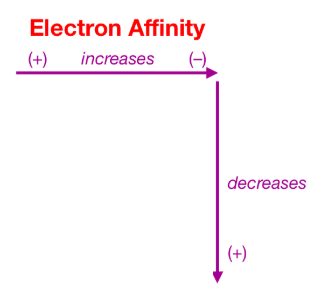# Solution: Of the following elements, __________ has the most negative electron affinity.A) NaB) LiC) BeD) NE) F

###### Problem

Of the following elements, __________ has the most negative electron affinity.

A) Na

B) Li

C) Be

D) N

E) F

###### Solution

We’re being asked to determine the element with the highest (most negative) electron affinity

Recall that electron affinity is the energy change from the addition of 1 e to a gaseous element/ion

It is represented by this chemical equation:

Atom(g) + e  Ion(g), ΔE = –E.A.

The trend for electron affinity is as follows: it increases from left to right and decreases down a period in the periodic table.The given elements are:View Complete Written Solution